Скачать презентацию Graph A diagram that exhibits a relationship often

d6f1f8794b50c629bd1ec92ab92aa9bd.ppt

• Количество слайдов: 13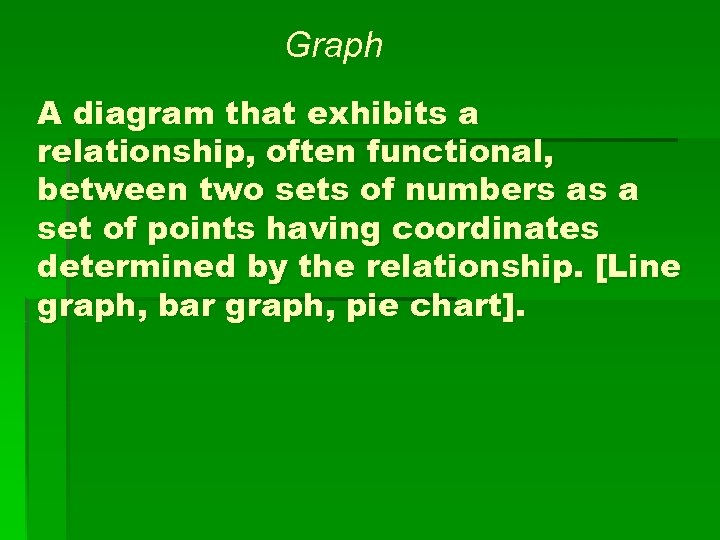Graph A diagram that exhibits a relationship, often functional, between two sets of numbers as a set of points having coordinates determined by the relationship. [Line graph, bar graph, pie chart].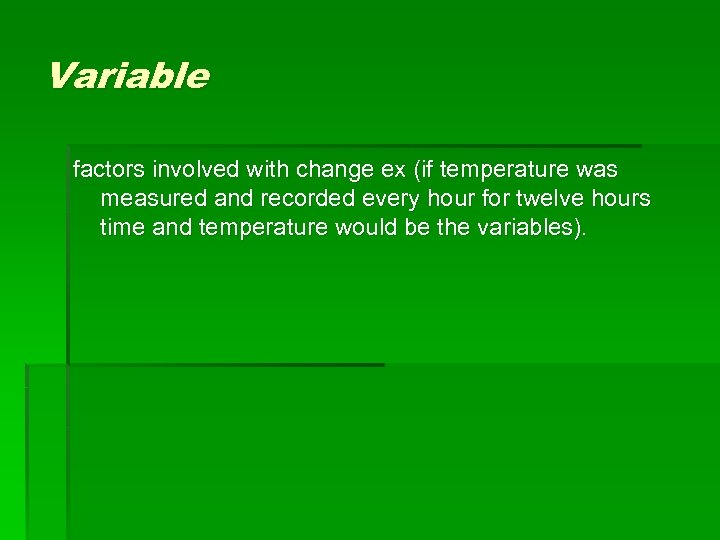Variable factors involved with change ex (if temperature was measured and recorded every hour for twelve hours time and temperature would be the variables).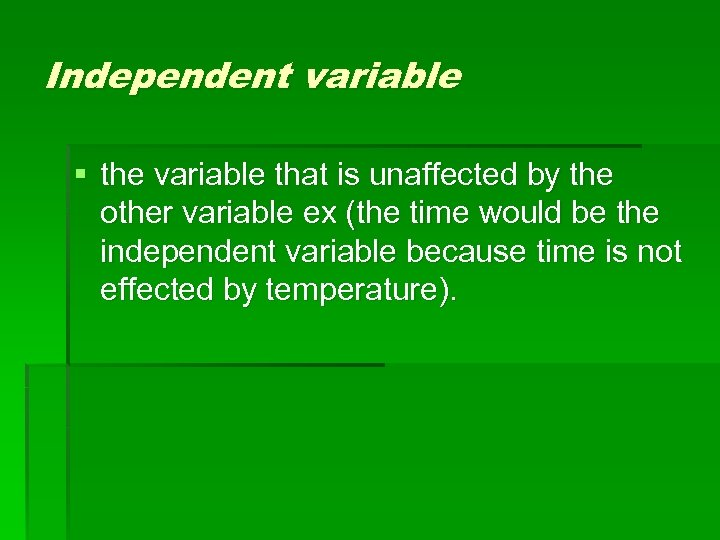Independent variable § the variable that is unaffected by the other variable ex (the time would be the independent variable because time is not effected by temperature).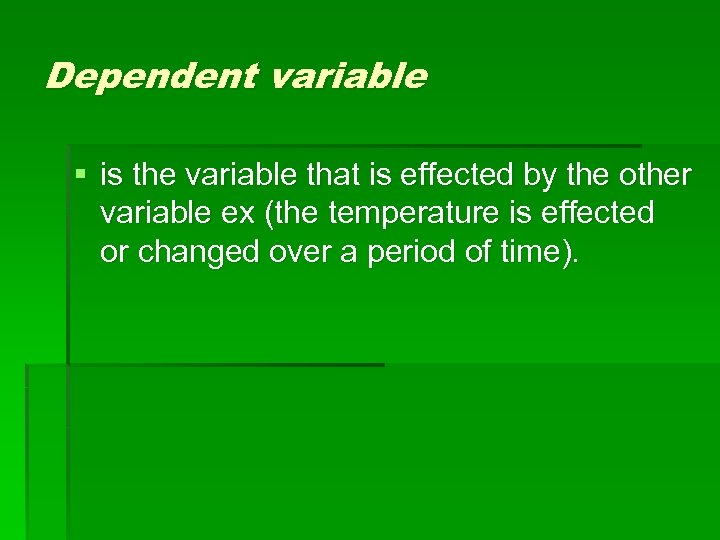Dependent variable § is the variable that is effected by the other variable ex (the temperature is effected or changed over a period of time).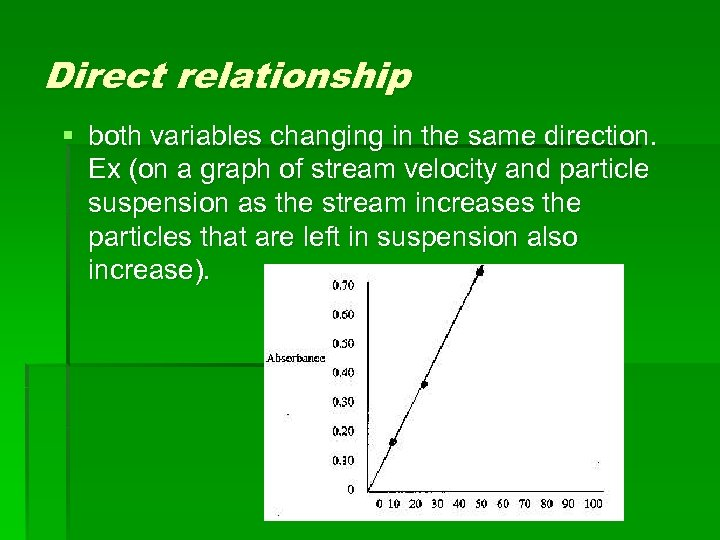Direct relationship § both variables changing in the same direction. Ex (on a graph of stream velocity and particle suspension as the stream increases the particles that are left in suspension also increase).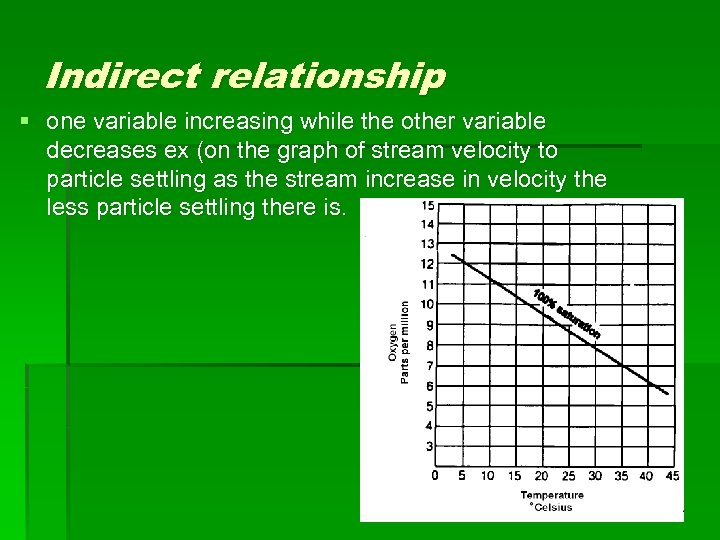Indirect relationship § one variable increasing while the other variable decreases ex (on the graph of stream velocity to particle settling as the stream increase in velocity the less particle settling there is.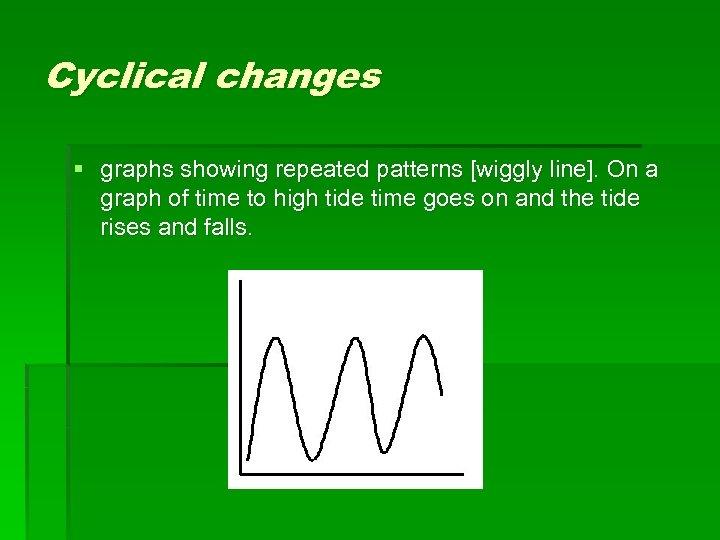Cyclical changes § graphs showing repeated patterns [wiggly line]. On a graph of time to high tide time goes on and the tide rises and falls.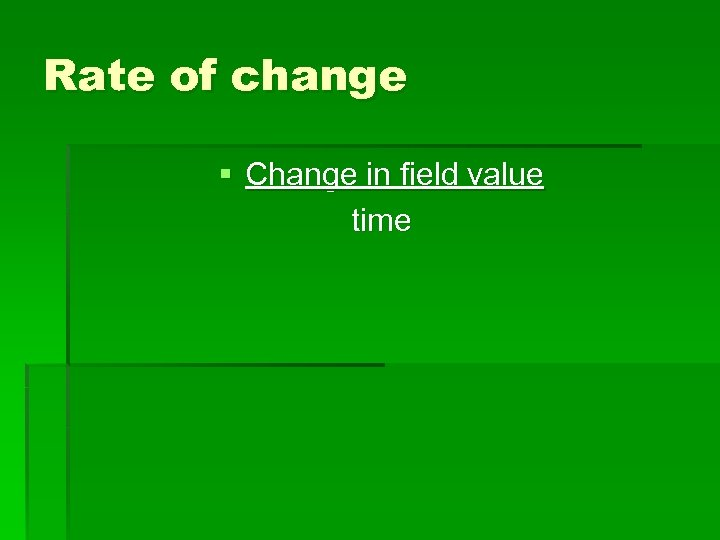Rate of change § Change in field value time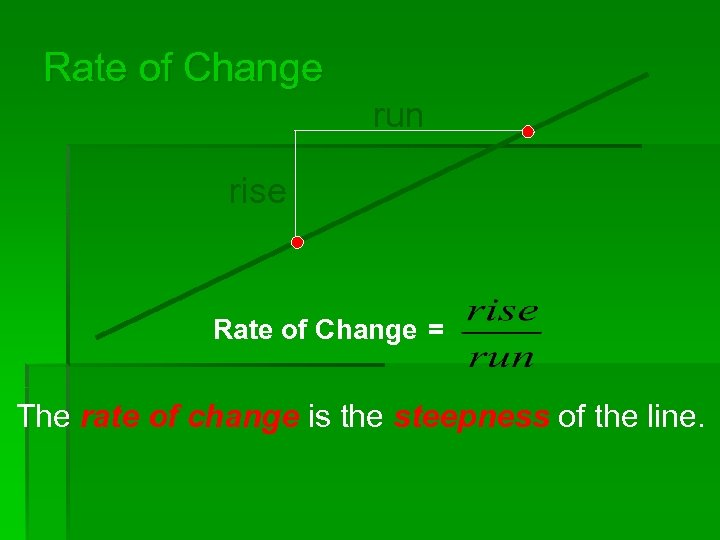Rate of Change run rise Rate of Change = The rate of change is the steepness of the line.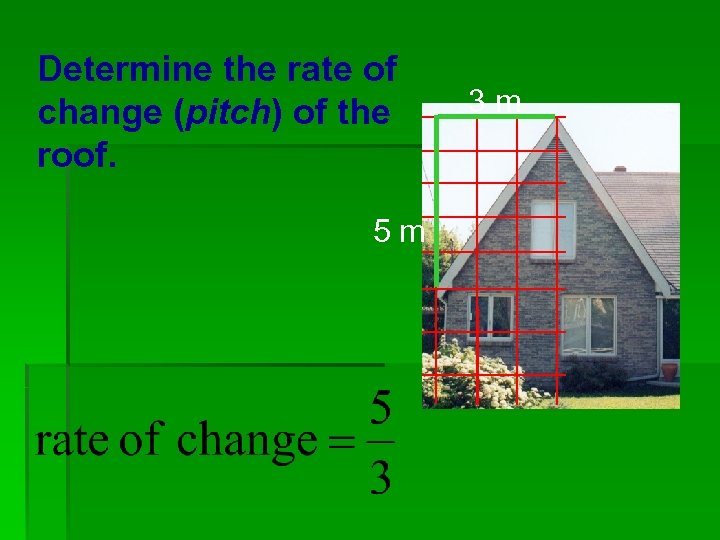Determine the rate of change (pitch) of the roof. 5 m 3 m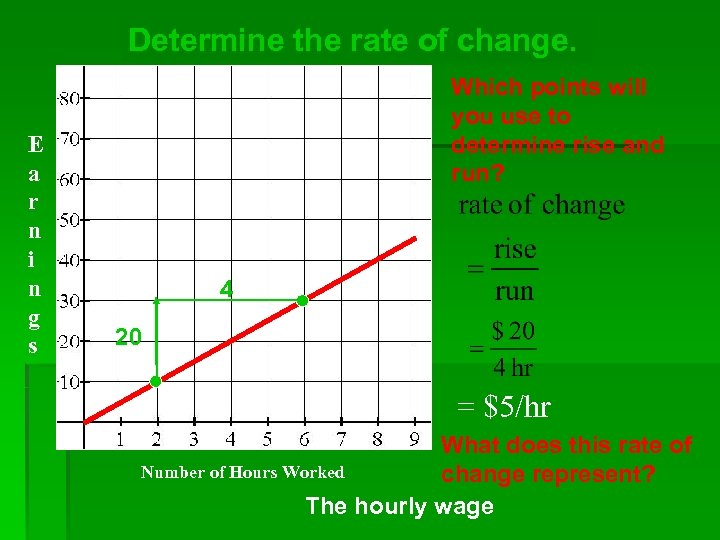Determine the rate of change. E a r n i n g s Which points will you use to determine rise and run? 4 20 = \$5/hr What does this rate of Number of Hours Worked change represent? The hourly wage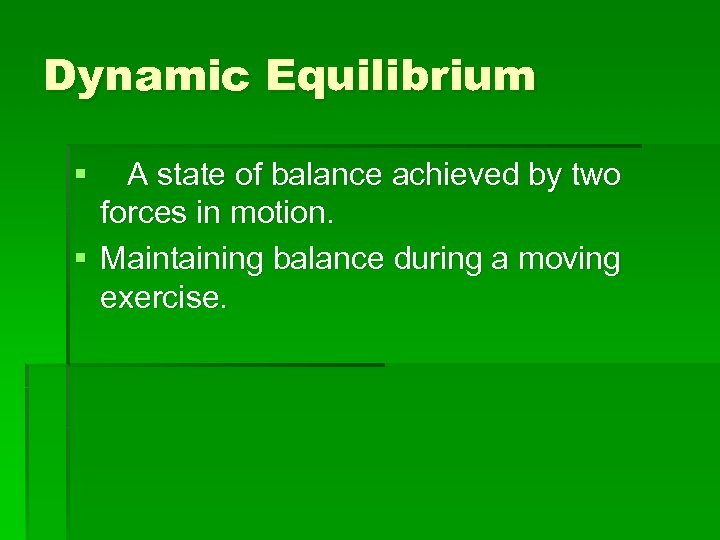Dynamic Equilibrium § A state of balance achieved by two forces in motion. § Maintaining balance during a moving exercise.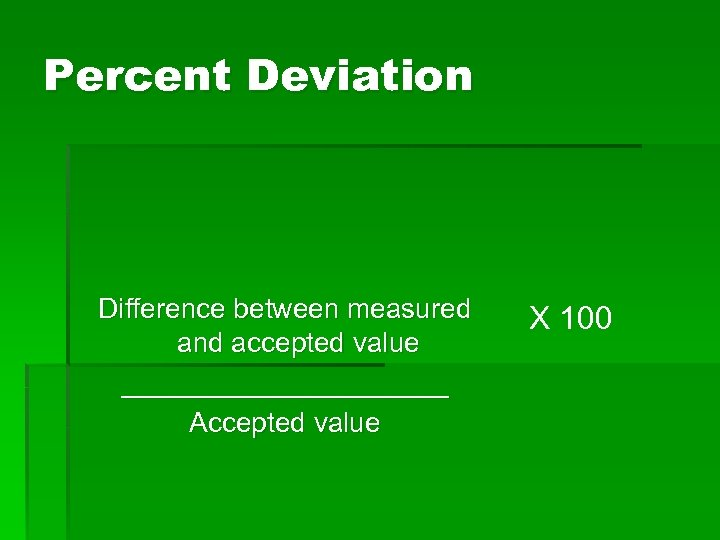Percent Deviation Difference between measured and accepted value ___________ Accepted value X 100